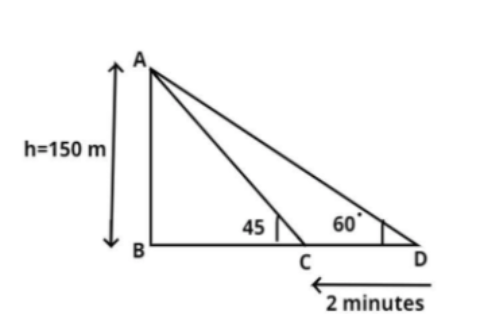Courses
Courses for Kids
Free study material
Free LIVE classes
MoreLIVE
Join Vedantu’s FREE Mastercalss

# A moving boat is observed from the top of a cliff of 150 m height. The angle of depression of the boat changes from ${60^0}$ to ${45^0}$ in 2 minutes. What is the speed of the boat in meters per hour?$a.{\text{ }}\dfrac{{4500}}{{\sqrt 3 }} \\ b.{\text{ }}\dfrac{{4500\left( {\sqrt 3 - 1} \right)}}{{\sqrt 3 }} \\ c.{\text{ 4500}}\sqrt 3 \\ d.{\text{ }}\dfrac{{4500\left( {\sqrt 3 + 1} \right)}}{{\sqrt 3 }} \\$Verified
270k+ views
Hint: in this question first calculate the distance BC and BD using property of $\left( {\tan \theta } \right)$ which is perpendicular divided by base (see figure), then calculate DC, then we know that speed is the ratio of distance to time, so use this concept to reach the solution of the question.It is given that the height (h) of the cliff is 150 m.
As we know that the cliff is up right so it makes a right angle with the base.
$\Rightarrow \angle ABD = {90^0}$
Now the angle of depression of the boat from top of the cliff is ${60^0}$ see figure, and this angle of depression changes from ${60^0}$ to ${45^0}$ in 2 minutes.
So, time taken to travel distance DC is equal to 2 minutes, now convert this time into hour.
So, as we know that $60{\text{ min = 1 hour}}$
$\Rightarrow 1\min = \dfrac{1}{{60}}hr \\ \Rightarrow 2\min = \dfrac{2}{{60}}hr = \dfrac{1}{{30}}hr \\$
So, time (t) taken to travel distance DC is equal to $\dfrac{1}{{30}}hr$.
$\Rightarrow t = \dfrac{1}{{30}}hr$
Now as we know that distance is equal to multiplication of speed and time.
$\Rightarrow {\text{speed = }}\dfrac{{{\text{Distance}}}}{{{\text{Time}}}}$………….. (1)
So, first calculate the distance DC.
Let, BD = x meter.
In triangle ABD
$\tan {60^0} = \dfrac{{{\text{AB}}}}{{{\text{BD}}}} = \dfrac{{150}}{x} \\ \Rightarrow x = \dfrac{{150}}{{\tan {{60}^0}}} = \dfrac{{150}}{{\sqrt 3 }} \\$
Now multiply by $\sqrt 3$in denominator and numerator we have,
$x = \dfrac{{150}}{{\sqrt 3 }}.\dfrac{{\sqrt 3 }}{{\sqrt 3 }} = \dfrac{{150\sqrt 3 }}{3} = 50\sqrt 3$meter.
Now, in triangle ABC
Let, BC = y meter
$\tan {45^0} = \dfrac{{{\text{AB}}}}{{{\text{BC}}}} = \dfrac{{150}}{y}$
$\Rightarrow y = \dfrac{{150}}{{\tan {{45}^0}}} = \dfrac{{150}}{1} = 150$ meter.
Now from figure we can say that,
${\text{DC = BD}} - {\text{ BC}}$
$\Rightarrow DC = x - y$
$\Rightarrow DC = 150 - 50\sqrt 3 = 150\left( {1 - \dfrac{{\sqrt 3 }}{3}} \right) = 150\left( {1 - \dfrac{1}{{\sqrt 3 }}} \right) = \dfrac{{150}}{{\sqrt 3 }}\left( {\sqrt 3 - 1} \right)$ meter.
Now from equation (1), we have
$\Rightarrow {\text{speed }}\left( s \right){\text{ = }}\dfrac{{{\text{Distance}}}}{{{\text{Time}}}}$
$\Rightarrow s = \dfrac{{DC}}{t}$
$\Rightarrow s = \dfrac{{\dfrac{{150}}{{\sqrt 3 }}\left( {\sqrt 3 - 1} \right)}}{{\dfrac{1}{{30}}}} = \dfrac{{\left( {150 \times 30} \right)\left( {\sqrt 3 - 1} \right)}}{{\sqrt 3 }} = 4500\left( {\dfrac{{\sqrt 3 - 1}}{{\sqrt 3 }}} \right)$ Meters/hour.
So, the required speed of the boat in meter per hour is $4500\left( {\dfrac{{\sqrt 3 - 1}}{{\sqrt 3 }}} \right)$
Hence, option (b) is correct.

Note: In such types of question first draw the pictorial representation of the given problem as above, it will give us a clear picture what we have to find out, then first convert the unit of given time into hour using unit conversion as above, then apply some basic trigonometric properties and calculate the distance DC in meter then according to the formula of speed, distance, and time calculate the speed of the boat in meters per hour.
Last updated date: 16th Sep 2023
Total views: 270k
Views today: 4.70k